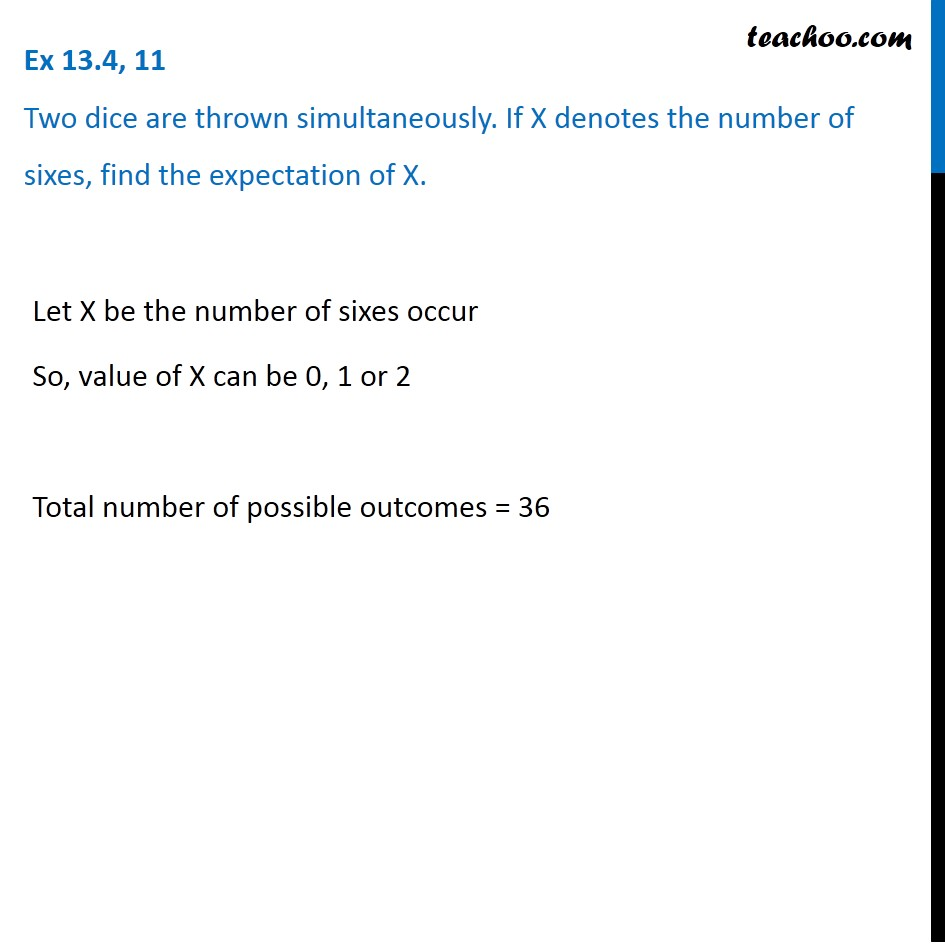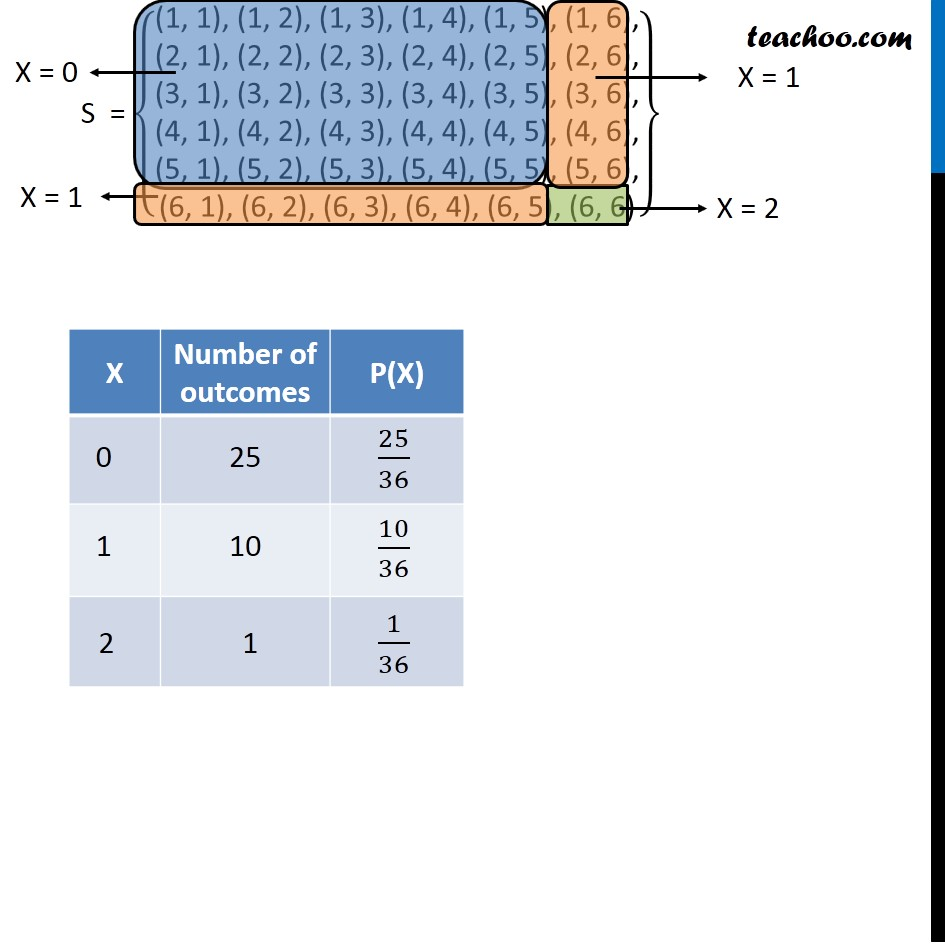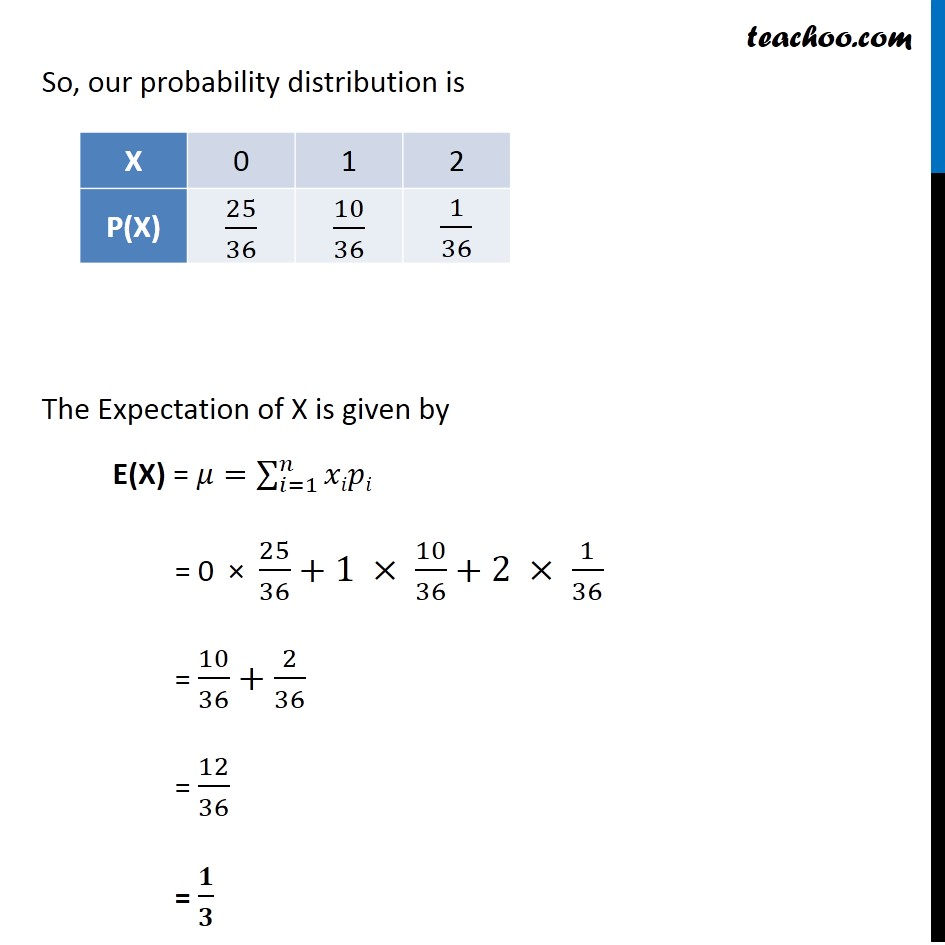Mean or Expectation of random variable

Chapter 13 Class 12 Probability (Term 2)
Concept wise### Transcript

Ex 13.4, 11 Two dice are thrown simultaneously. If X denotes the number of sixes, find the expectation of X.Let X be the number of sixes occur So, value of X can be 0, 1 or 2 Total number of possible outcomes = 36 So, our probability distribution is The Expectation of X is given by E(X) = 𝜇=∑_(𝑖=1)^𝑛▒𝑥𝑖𝑝𝑖 = 0 × 25/36+1 × 10/36+2 × 1/36 = 10/36+2/36 = 12/36 = 𝟏/𝟑